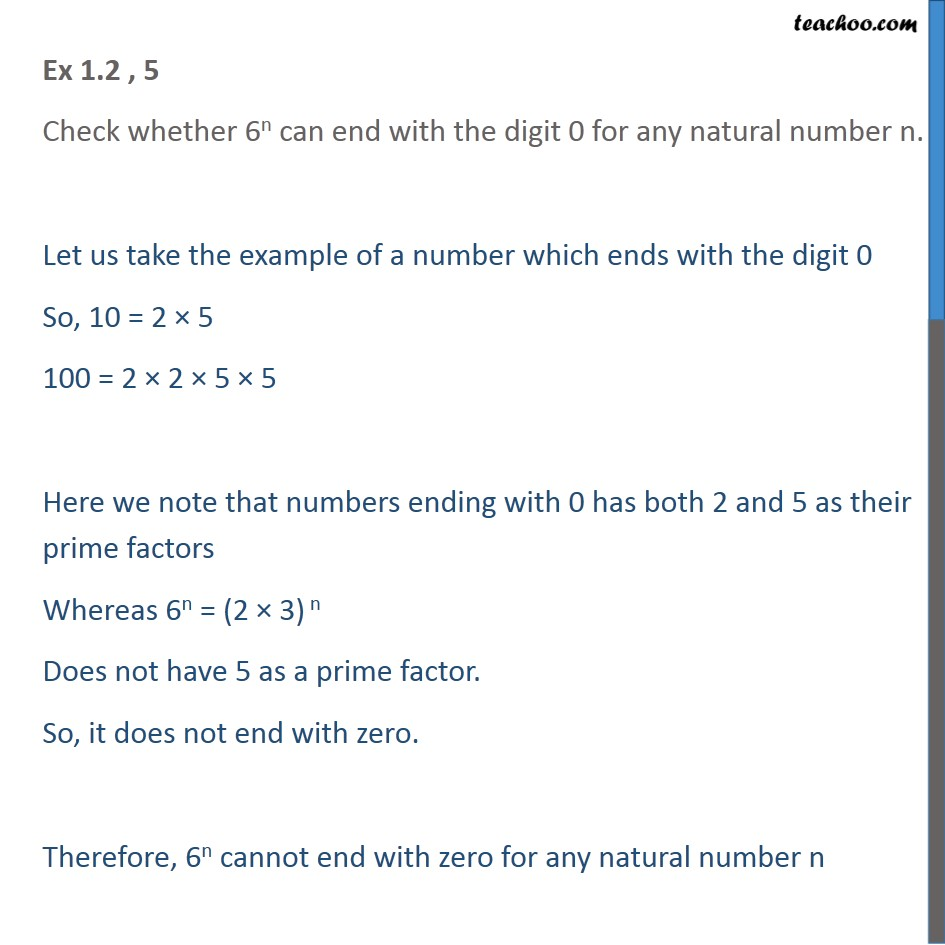Check Important Questions and Chapter Summary -https://you.tube/Real-Numbers-Class-101. Chapter 1 Class 10 Real Numbers
2. Concept wise
3. Prime Factorization

Transcript

Ex 1.2 , 5 Check whether 6n can end with the digit 0 for any natural number n. Let us take the example of a number which ends with the digit 0 So, 10 = 2 5 100 = 2 2 5 5 Here we note that numbers ending with 0 has both 2 and 5 as their prime factors Whereas 6n = (2 3) n Does not have 5 as a prime factor. So, it does not end with zero. Therefore, 6n cannot end with zero for any natural number n

Prime Factorization